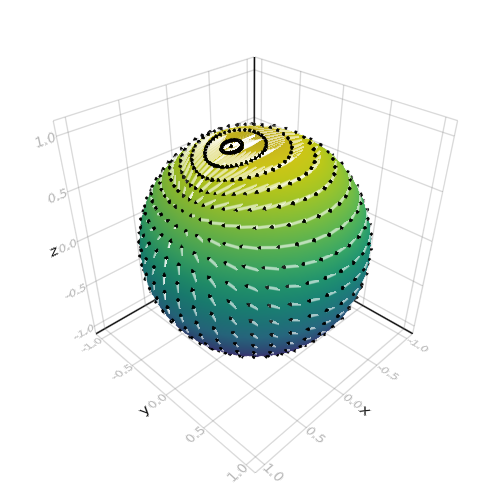## Arrows on Sphere

```
using LinearAlgebra

using AbstractPlotting

n = 20
f   = (x,y,z) -> x*exp(cos(y)*z)
∇f  = (x,y,z) -> Point3f0(exp(cos(y)*z), -sin(y)*z*x*exp(cos(y)*z), x*cos(y)*exp(cos(y)*z))
∇ˢf = (x,y,z) -> ∇f(x,y,z) - Point3f0(x,y,z)*dot(Point3f0(x,y,z), ∇f(x,y,z))

θ = [0;(0.5:n-0.5)/n;1]
φ = [(0:2n-2)*2/(2n-1);2]
x = [cospi(φ)*sinpi(θ) for θ in θ, φ in φ]
y = [sinpi(φ)*sinpi(θ) for θ in θ, φ in φ]
z = [cospi(θ) for θ in θ, φ in φ]

pts = vec(Point3f0.(x, y, z))
∇ˢF = vec(∇ˢf.(x, y, z)) .* 0.1f0
surface(x, y, z)
arrows!(
pts, ∇ˢF,
arrowsize = 0.03, linecolor = (:white, 0.6), linewidth = 3
)

```